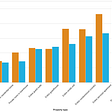# Properties of a Statistic

Sufficiency, Robustness, and more

Please see post1 for an introduction to statistical modeling and post2 to understand the difference between a statistic and an estimator.

Let’s revisit what is a statistic?

A statistic is a single value that is calculated from the sample data.

What is it used for?

Well, as the definition suggests, it is used to describe a sample of data besides estimating a population parameter or evaluating a hypothesis.

Why do we need to study it?

We need a statistic of the sample data in order to understand the characteristics of the entire population, as it is not feasible to study the entire population.

Hence, we need to be apprised of its various properties. For example, mean is an unbiased estimator of the population mean, where ‘unbiased’ is the property of a statistic.

So, now that we have a good understanding of what is a statistic, sample, and population data, let us learn about various properties of a statistic:

1) Completeness: It is a property of a statistic to ensure that different values of the parameters lead to different distributions. It is similar to the concept of “Identifiability”.

Definition:

Let’s assume the following:

T: statistic

X: random variable

: parametrized model

θ: parameter

g: measurable function

Then, a statistic T is boundedly complete for the distribution of X if the following holds true for every measurable function g (that is also bounded):

2) Sufficiency: It is a property that refers to the use of all the information that could be derived from a sample to estimate the corresponding parameter.

In other words, there does not exist any other statistic that could provide more information about the parameter value, when calculated from the same sample.

It is a function of data X that contains all the information needed to arrive at the estimate. It could be as simple as taking the sum of all the data points.

There are cases where sufficient statistic is a set of functions called as “jointly sufficient statistic”.

Jointly sufficient statistic of a Gaussian distribution (with unknown mean and variance) estimates both the parameters:

• the sum of all data points called the sample mean
• the sum of all squared data points called the sample variance

Note that mean is a sufficient statistic as it uses all the data, but median and mode are not very sufficient as they do not use entire data points

Definition:

A statistic T is sufficient for underlying parameter θ if the conditional probability distribution of the data X does not depend on the parameter, given the statistic t = T(X)

3) Unbiasedness:

It is a measure of how close a sample statistic is to its corresponding population parameter.

Definition:

Let’s assume the following:

T: statistic

Θ: True parameter

E(T): the expected value of the statistic T, then:

Where: bias(θ) is the bias of the statistic T

Note that if bias(θ) = 0, then the expected value of the statistic T becomes equal to its true value, hence T is an unbiased estimator of θ

Let us look at some of the common types of bias:

• Selection bias: When a certain group of individuals is selected more often than the others leading to the bias in collecting the sample data.
• Reporting bias: When a certain type of observations is more likely to be reported, it induces reporting bias
• Omitted-variable bias: The parameter estimation becomes biased in regression analysis when an independent variable is omitted from the model due to a certain assumption
• Detection bias: When you look for a particular behavior more often in a certain group, it is more likely to get detected among them. For example, Diabetes is more likely to be detected in a group of patients that are obese.

4) Efficiency: It is a measure of the change in the statistic from one sample to another. It is characterized by a small variance, signaling the small deviation between the estimated and true value of the parameter.

Let’s take two unbiased estimators T₁ and T₂ for some parameter θ, such that Var(T₁) > Var(T₂). Therefore, T₁ is less efficient than T₂.

If X is a sample mean of n iid random variables X₁, X₂…etc with the population mean, 𝜇. Now, if we take infinite repeated samples from such distribution, we observe that mean of each sample would be close to population mean 𝜇. Also, the mean of such sample means would be 𝜇. This is because the probability of occurrence of samples far off from the center of such distribution is very less. Thus, most repeated samples were expected to have a value closer to the center i.e. 𝜇.

5) Robustness: A statistic is considered to be robust if it is not overly affected by the outliers or some deviations in model assumptions. That implies if assumptions are only met reasonably, the statistics will still have a reasonable efficiency and a small bias.

How to check the robustness? Add an outlier to the dataset and check what happens to the estimator vs when the outlier is replaced with one of the existing data points. Repeating such an experiment for multiple additions and replacements will reveal how robust the estimator is.

Let’s understand this with an example:

Dataset, D: {1, 12, 8, 6 ,19}, Mean = 9.2, Median = 8

If we add +1000, D: {1, 12, 8, 6 ,19, 1000}, Mean = 174.3, Median = 10

We can see from the example above that mean of the new data is very different from the mean of the original data, whereas the median of the two datasets is relatively similar. Hence, the median is a robust measure whereas the mean is not.

With this, we have reached the end of the article. We learned about the various properties of a statistic.

Happy learning!!!

References:

--

--

--

## More from WiCDS

A collaborative community for Women in Data Science and Programming to learn and grow

## Introduction to PowerBI and Get started with PowerBI, Prepare data for analysis and Model data in…## Text Mining for Dummies: Text Classification with Python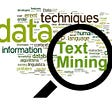## Case Study based discussion on performance parameters of classification, regression, and clustering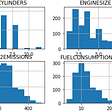## Finding the Safest Store to Shop During COVID Outbreak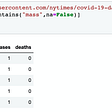## A Multi Clustered approach for Predicting Covid-19 Spread## Guide to implement Linear Regression in Pyspark and R## Python V/S R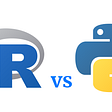Data Scientist

## Fraud Prevention: Exploration-Exploitation Tradeoff in AI-based Systems## Are we there yet? The First-Mile and Last-Mile problem with Machine Learning Models## How much is YOUR property worth on Airbnb?